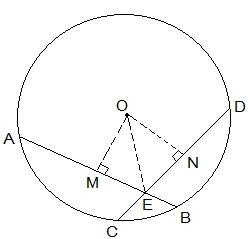Guru

# If two equal chords of a circle intersect within the circle, prove that the segments of one chord are equal to corresponding segments of the other chord. Q.2

• 0

How i solve the question of class 9th ncert math of Circles chapter of exercise 10.1of question no (2). I think it is very important question of class 9th give me the tricky way for solving this question If two equal chords of a circle intersect within the circle, prove that the segments of one chord are equal to corresponding segments of the other chord.

Share

1. This answer was edited.

Let AB and CD be two equal cords (i.e. AB = CD). In the above question, it is given that AB and CD intersect at a point, say, E.

It is now to be proven that the line segments AE = DE and CE = BE

Construction Steps:

Step 1: From the center of the circle, draw a perpendicular to AB i.e. OM ⊥ AB

Step 2: Similarly, draw ON ⊥ CD.

Step 3: Join OE.

Now, the diagram is as follows-Proof:

From the diagram, it is seen that OM bisects AB and so, OM ⊥ AB

Similarly, ON bisects CD and so, ON ⊥ CD

It is known that AB = CD. So,

AM = ND — (i)

and MB = CN — (ii)

Now, triangles ΔOME and ΔONE are similar by RHS congruency since

OME = ONE (They are perpendiculars)

OE = OE (It is the common side)

OM = ON (AB and CD are equal and so, they are equidistant from the centre)

∴ ΔOME ΔONE

ME = EN (by CPCT) — (iii)

Now, from equations (i) and (ii) we get,

AM+ME = ND+EN

So, AE = ED

Now from equations (ii) and (iii) we get,

MB-ME = CN-EN

So, EB = CE (Hence proved).

• 0